# Instruction for the Seismic fast version

Dear Jason,

Thank you very much for your quick answer. I will first try to find the frequencies with the linearization and the eigenalalysis, then with the BModes software.
For the the linearization, I’m using the exemple 1 from the seismic archive. I have found a steady state solution (in region 2, with the rotor speed of 12,1 rad/s), and the linearization is made without control inputs and with only a horizontal hub-height wind speed disturbance. Then when I use the lin file created to get data to use the Campbell Diagram, the natural frequencies which are given are the following ones (hence my Campbell excel file is totally wrong) :

0
3,9512
3,0558
3,0979
2,1885
2,0494
1,7977
1,7083
1,2931
0,8876
0,9014
0,7366
0,5777
0,4133
0,3975
0,0076
0,0017
1,742
0,000
5,7839

I don’t understand why this values are not the same as some I should definitely find for the 5 MW wind turbine. I have tried to reduce the number of DOFs without success. Do you have a typical working file for this or any advices ? I probably miss something but I can’t find any solutions.

Thank you very much one more time for your help…

Pierre-Yves

Dear Pierre-Yves,

I’m not sure your results are totally wrong. I see frequencies that are close to those expected for the tower and blades. I do see two sets of rigid-body modes with pairs of zero (or near-zero) frequency; I would normally expect to only see one associated with the rigid-body rotation of the drivetrain, but perhaps the second rigid-body mode has something to do with a linearization using the Seismic version of FAST and platform DOFs that are enabled? I’m not sure I can comment further without knowing more about your simulation set-up.

Best regards,

Dear Jason,

Thank you for your last answer ! I have nearly solved the issues of the frequencies. And I’m taking every day more control of the software. However, some results are still surprising. I tried to compare the results with a FEM of the NREL 5 MW wind turbine made on Matlab (I wanted to reproduce the Matlab results with Fast, a simulation with a idling turbine (rot speed=0), without wind conditions and with only an X direction earthquake motion), and it seems that there is a difference in the damping. After the earthquake, the structure should evolve with free oscillations, with a damping of 1%. Thank to Fourier transform, we see that the main frequency is as expected 0,328 Hz . And for this frequency, if we select only the free oscillations after the earthquake, we can see on the joined figure that the damping is more around 0.4 than 1%. Do you know where does this difference can come ? It’s really hard to reproduce results from papers (or obtained with a reliable model made with Matlab) in order to start personal analysis on solid bases.

Thank you very much for your help !!
Best regards,

Pierre-Yves

Dear Pierre,

I’m sorry, but I’m not really sure I understand your question or how to interpret your plot (what is the red curve?). Why are you expecting to see 1% damping?

Best regards,

Dear Jason,
Sorry there is a mistake in my figure. The blue curve are the free oscillations of the tower after the earthquake, the green one the exponential decrease for a 0.4% damping, and the red one for a 1% damping (for f = 0,328 Hz). Shouldn’t I expect to see 1% damping, if a set the tower mode structural damping ratios to 1% ?

Pierre-Yves

Dear Pierre-Yves,

OK, now I understand. Regarding the drop in tower damping from 1% to 0.4%, this is discussed in the following forum topic: Natural frequency and damping ratio calculation - #12 by Jason.Jonkman. But basically, the 1% damping ratio is specified for the isolated cantilevered tower, but when the tower is coupled to the full system, the overall damping level will change.

Best regards,

Dear Jason,

Thank you for your answer, I understand better the damping computation and how I can change them. Sorry for all the help I ask your for, but I have one last thing which annoys me. I was trying to understand why my response tend to be different with my FEM on Matlab, so I’ve made a free vibration test of the 5MW turbine.

The wind load is not considered. Yaw is disabled, rotor speed is 0. The base is fixed, no platform motion.

First fore-aft motion

Only the first fore-aft mode is enabled, all other modes are disabled. The initial displacement at the tower top is 0.5m. Damping ratio for the first fore-aft tower mode is 2% of critical. The displacement response is shown in Figure 1. Only a section of the response is shown here. Towards the end, the displacement oscillates about -0.015m level. It would be expected for the displacement to oscillate about 0 level. If we consider the small displacement due to eccentric mass of the rotor, the displacement should be positive. It is not clear why there is this residual negative displacement. When running seismic analysis with 0 initial displacement, the simulated result has a small negative initial displacement, which is also unexpected. It is not clear if the simulation considers the displacement due to gravity loads as the initial condition for simulating seismic response.

Figure 1.

The Fourier Amplitude of displacement is shown in Figure 2. The peak of the spectrum is at 0.327 Hz which is close to the first fore-aft frequency of the tower, as expected.

Figure 2.
From the computed response, by using the logarithmic decay method, the damping ratio was computed to be 0.75%. This is lower than the uncoupled tower fore-aft first mode damping ratio, which is due to inertia of the nacelle and rotor.

Figure 3 compares the FAST results with a SDOF response with the damping and frequency estimated from the FAST results. The comparison shows that the FAST results matches SDOF response relatively well. However, it is strangely overshoots the negative peaks, but matches the positive peaks. This is also an indication of the negative residual motion at the end of simulation as discussed above.

Figure 3

Second fore-aft mode:
Same analysis as before but only the second fore-aft mode enabled.
The Fourier Amplitude of the response is shown in Figure 4. It shows multiple peaks, which means that the motion is not harmonic, but contains multiple frequencies, or the frequency is changing in time. The largest peak is at 2.278 Hz, which corresponds to the second fore-aft frequency of the tower. The damping ratio is estimated to be 1.5%. This is twice the damping ratio in the first mode, although same value of 2% was used in FAST for the uncoupled tower. It appears that the difference between the tower damping ratio and the system damping ratio is higher for the first mode, but lower for higher modes, which is expected because of lower mass participation factors of higher modes.

Figure 4
The comparison between the FAST result and an equivalent SDOF is shown in Figure 5. The SDOF frequency is set to 2.13 Hz (different from the second mode frequency of 2.28 Hz) to match the first few peaks of the FAST response. It appears that after a few cycles, the FAST response lags behind the SDOF solution, and at some time the lag is so large that the two responses are out of phase.

Figure 5
The time-frequency plot of the FAST response and equivalent SDOF is shown in Figure 6. The results indicate that as time passes the frequency of FAST response decreases, and the motion is not harmonic as expected. This was unlike the first mode response which was found to be harmonic.

(a) FAST
(b) Harmonic
Figure 6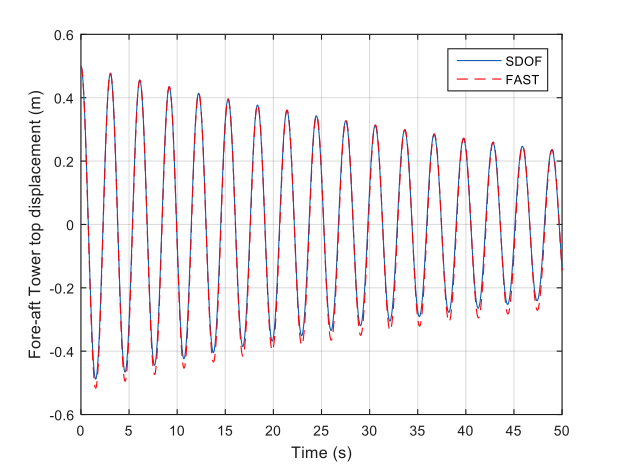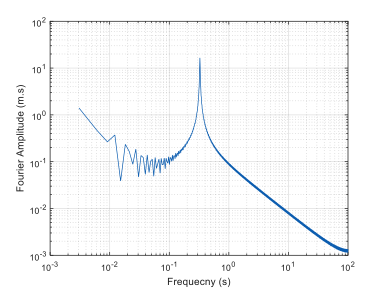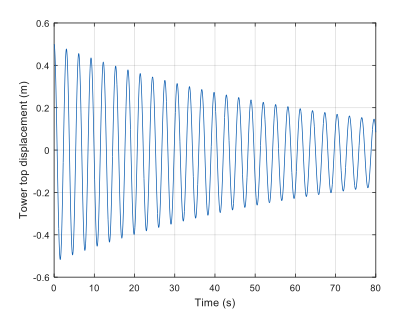The last figures. I think that solving this dephasing issue may be really useful !

One more time thank you very much for your help !

Best regards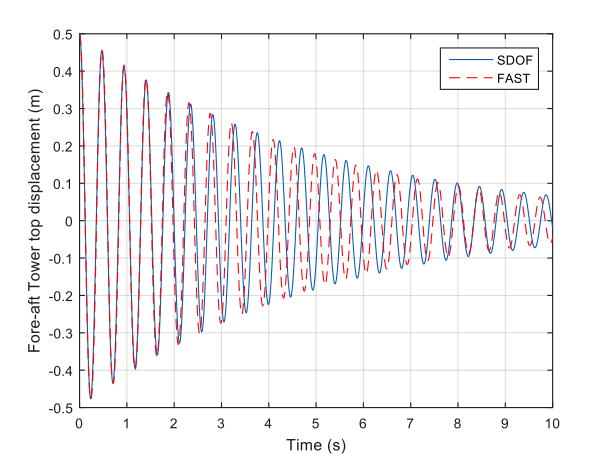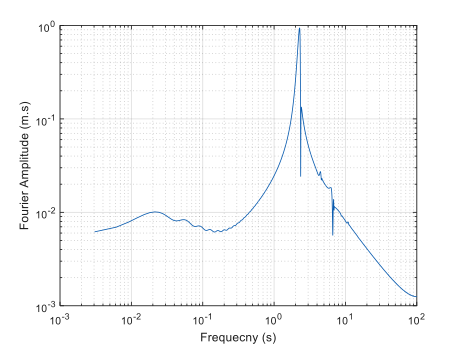Dear Pierre,

1. The negative mean displacement is expected. The tower displacement in FAST is defined as positive in the nominally downwind direction, but the overhanging weight of an upwind rotor will cause the tower to displace slightly upwind in the absence of aerodynamic loads.
2. For the simulation with only the second fore-aft bending mode enabled, a tower-top displacement of 0.5 m is very large (normally I would expect much less excitation/displacement of the second modes than the first), so, I would guess the nonlinearities in the response are likely the result of geometric nonlinear terms (e.g. the axial shortening) that are modeled in FAST. The geometric nonlinear terms will have a smaller effect for smaller displacements.
I hope that helps.

Best regards,

Hi Jason,

are there any plans to include a/the seismic modul in the latest version of OpenFAST?

Best regards,
Simon

Dear Simon,

That would be great, but NREL has not yet had the funding to do that.

Best regards,

Dear Jason,

Is there a way I can add the already existing seismic load code in FASTv8 or open fast and recompile the same? I understand that the seismic forces required to achieve the user motions are calculated at every step. I believe it would reasonably be not so difficult. But, I need to know which module do I need to focus on changing? It would be great if you could give a brief idea of where I can supply these force calculations at the base node in the source code.
I eagerly want to stop using the seismic module of FAST v7 and utilize the upgraded capabilities in the higher version. Please help me. Thanks.

Dear @Kashyap.Subham,

It is not possible without modification of the source code to interface the Seismic routines from FAST v7 into OpenFAST. And again, this is not something NREL has been funded to do yet.

That said, if you know the time-series of seismic motion as computed by the Seismic routines from FAST v7, you can use these within OpenFAST by developing a simple `ExtPtfm` routine available with `CompSub` = 2, e.g., as discussed in the following OpenFAST issue: Develop Prescribed Platform Motion capability with aero-elasticity · Issue #617 · OpenFAST/openfast · GitHub. But this simple approach cannot be combined with the offshore functionality of OpenFAST (with HydroDyn, SubDyn ) for combined wind + wave + seismic loading because the `CompSub` = 2 option is not available when SubDyn is enabled (which is `CompSub` = 1).

Best regards,

1 Like

Dear Sir,

I wish to implement the seismic scenario in FAST v7 but with distributed springs. I understand that this cannot be done without modifying the source code of FAST v7. I can see that subroutines for FAST v7 DS and Seismic are available on the forum. Is there a way I can combine the two? I would really like your help and guidance in changing the source code for the same. I request you to help me get started with it.
I have explored your options for seismic implementation in openfast using extptfm but, I am not at the disposal of using new software at this stage to generate superelements though it really sounds more promising. I am only left with the option to change the source code.

What I understand related to the code is as follows:

1. The foundation elements along with their properties need to be added to the structural model.
2. At each soil layer, I need to define a soil curve that as well interpolates the forces if the nonlinear behavior of the soil has to be simulated.
3. The ground motion has to be taken input at the base of the pile.
4. The superstructure force (above the seabed) will allow for deformations of the soil springs.
5. Using the soil curve, the reaction forces at each pile-spring interface need to be added to the equation of motion.

Please correct me if I am wrong. In addition, though I know the procedure somehow, I am unaware of the subroutines to be changed to bring about these changes. Kindly advise.

Thanks
Subham

Dear @Kashyap.Subham,

At one point we developed a customized version of FAST v7 that supported distributed springs (DS) modeling of the foundation. This is discussed in the following forum topic: Turbine-soil interaction. Influence on the mode shapes., especially my post dated Feb 01, 2013, where I share an example UserTwrLd_DS.f90 file.

This routine has not been developed to include seismic excitation, but should hopefully be a good start to that. Because I have not done what you want myself, I cannot provide detailed guidance, but to answer your specific questions:

1. Agreed. The FAST v7 model that makes use of `UserTwrLd_DS.f90` places the platform reference point at the bottom of the pile (where 6 DOF motion is available), and the entire pile + tower is modeled via the tower element in FAST v7.
2. The UserTwrLd_DS.f90 file implements linear springs at each node along the pile, but this could be changed to model nonlinear springs.
3. I would not expect to that you’d apply ground motion only at the base of pile. Rather, I would expect that the deflection of each spring distributed along the pile would be the difference between the actual deformation of the pile and the position defined by the seismic event.
4. I’m not sure what you mean, but I would not expect that anything in the source code would need to be changed apart from the UserTwrLd_DS.f90 file.
5. This will be done automatically by FAST v7. It is just up to you define the loads to be applied distributed along the pile in the UserTwrLd_DS.f90 file.

Best regards,

For a general MDOF system, the equation of motion with base excitation can be given as follows:

[M]{xddot} + [C]{xdot} + [K]{x} = -[M]{xgddot}[U]

where x represents the relative structural displacement, and [U] is a column vector consisting of 1 or 0 as per the dofs in translation with the earthquake.

The above equation of motion is a general representation of seismic simulation as in textbooks.

As per my understanding, there will be additional resisting forces due to the flexible soil foundation. These forces as well need to be added to the above equation of motion at the nodes where the soil springs are introduced.

1. How do I identify these nodes in the DS subroutine? Let’s assume I just want to implement the seismic load case with the linear springs, to begin with.

2. How do I estimate the right-hand side of the equation in FAST v7 DS routine?

3. The soil reaction forces shall be added as an external force at each time step to evaluate the net deformations. What parameters shall I be looking at?

These are some basic queries. I hope I am making some sense of this. Request you to clarify and advise.

Subham

Subham

Dear @Kashyap.Subham,

If I understand your equation, this is equivalent to the following (changing notation a bit):

[M]{xsdd} + [C]{xsd} + [K]{xs} = [C]{xgd} + [K]{xg}

where xs is the structural displacement and xg is the ground motion. This is the equation used by the Seismic module of FAST v7. Given the equation [M]{xsdd} = {f}, The user-defined platform (`UserPtfmLd`) and tower loading routines (`UserTwrLd`) in FAST v7 expect that you’ll provide a force {f} applied to the platform reference point for `UserPtfmLd` or each tower analysis node for `UserTwrLd`. Rearranging the equation above, this implies that:

{f} = [C]{xgd-xsd} + [K]{xg-xs}

1. See above. The `UserTwrLd` routine in FAST v7 will be called once for each tower analysis node. There is logic already within UserTwrLd_DS.f90 to ensure that loads are only applied to nodes below the seabed.
2. See above.
3. I’m not sure I understand what you are asking, but assessing the motions of the tower analysis nodes and structural reactions at the tower analysis nodes sounds important.

Best regards,

1 Like

Dear Sir,

It seems the most important line of the code in UserTwrLd for distributed spring is the following:
TwrFt(K) = TwrFt(K) - Stff(JNode)*X(K)*DZFract

I believe this corresponds to the force calculation you wrote as:
{f} = [C]{xgd-xsd} + [K]{xg-xs},

but, obviously without the earthquake-related variables. If my understanding is correct; I need to add the following extra terms in the first equation for the damping and stiffness terms as:

TwrFt(K) = TwrFt(K) - Stff(JNode)X(K)DZFract + (Dmp(JNode){xgd} + Stff(JNode){xg})

Is this legit? I believe the major task would be to establish ground motions at each spring location describing its velocity and displacement time series. (i.e., for a linear stiffness as already implemented).

Does this all sound valid?

Thanks
Subham

Dear @Kashyap.Subham,

I agree, except that:

• You should also subtract `Dmp(JNode)*XD(K)` from the right-hand side
• You should multiply all stiffness and damping terms (not just `Stff(JNode)*X(K)`) by `DZFract`

Best regards,

1 Like

Dear Sir,

I would request you to link all the source code files and associated libraries required to recompile FAST v7 for the tentative completion of this conversation. It would be great if you can also, attach the document guidance on the compilation of FAST v7. It shall serve as a good checkpoint for me.

Thanks
Subham